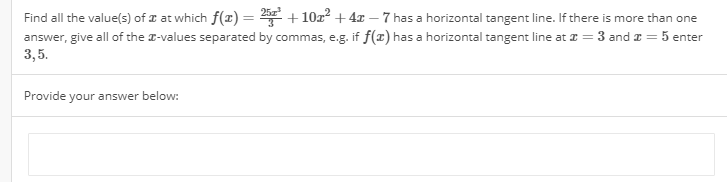# Find all the valuels) of z at which f(102 4z - 7 has a horizontal tangent line. If there is more than oneanswer, give all of the Z-values separated by commas, eg. if f(z) has a horizontal tangent line at z = 3 and z = 5 enter3,5.Provide your answer below:

Question
23 viewshelp_outlineImage TranscriptioncloseFind all the valuels) of z at which f(102 4z - 7 has a horizontal tangent line. If there is more than one answer, give all of the Z-values separated by commas, eg. if f(z) has a horizontal tangent line at z = 3 and z = 5 enter 3,5. Provide your answer below: fullscreen
check_circle

Step 1

Tangent line is horizontal means slope = f'(x) = 0.

Find the first derivative of the given function as follows.

Step 2

Equate the first derivative to zero to find the points wh...

### Want to see the full answer?

See Solution

#### Want to see this answer and more?

Solutions are written by subject experts who are available 24/7. Questions are typically answered within 1 hour.*

See Solution
*Response times may vary by subject and question.
Tagged in

### Functions